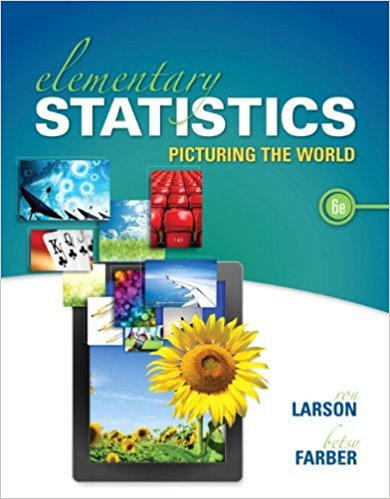×
Get Full Access to Elementary Statistics: Picturing The World - 6 Edition - Chapter 3.1 - Problem 16e
Get Full Access to Elementary Statistics: Picturing The World - 6 Edition - Chapter 3.1 - Problem 16e

×

# Solved: Identifying a Sample Space In Exercise, identifyISBN: 9780321911216 66

## Solution for problem 16E Chapter 3.1

Elementary Statistics: Picturing the World | 6th Edition

• Textbook Solutions
• 2901 Step-by-step solutions solved by professors and subject experts
• Get 24/7 help from StudySoup virtual teaching assistantsElementary Statistics: Picturing the World | 6th Edition

4 5 1 410 Reviews
29
5
Problem 16E

Problem 16E

Identifying a Sample Space In Exercise, identify the sample space of the probability experiment and determine the number of outcomes in the sample space. Draw a tree diagram when appropriate.

Guessing a student’s letter grade (A, B, C, D, F) in a class

Step-by-Step Solution:
Step 1 of 3

Solution 16E

Step1 of 2:

From the given problem we are guessing a student’s letter grade (A, B, C, D, F) in a class.

We need to identify the sample space of the probability experiment and determine the number of outcomes in the sample space.

Step2 of 2:

The sample is guessing a student’s letter grade and it is given below:

Sample space = {A, B, C, D, F}

The outcome is 5.

Step 2 of 3

Step 3 of 3

##### ISBN: 9780321911216

Unlock Textbook Solution